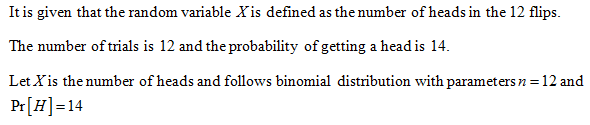# Rework problem 13 from section 4.3 of your text, involving the expected number of heads when flipping a coin. Assume that the coin is weighted so that Pr[H]=14, and that it is flipped 12 times. The random variable X is defined as the number of heads in the 12 flips.What is E[X]?

Question
84 views

Rework problem 13 from section 4.3 of your text, involving the expected number of heads when flipping a coin. Assume that the coin is weighted so that Pr[H]=14, and that it is flipped 12 times. The random variable X is defined as the number of heads in the 12 flips.

What is E[X]?

check_circle

Step 1...

### Want to see the full answer?

See Solution

#### Want to see this answer and more?

Solutions are written by subject experts who are available 24/7. Questions are typically answered within 1 hour.*

See Solution
*Response times may vary by subject and question.
Tagged in